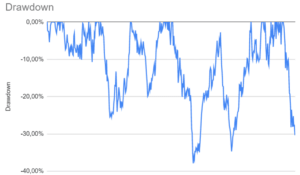# Conditional drawdown at risk (CDAR)

Conditional drawdown at risk (CDAR) at risk is very similar to conditional value-at-risk. Both metrics measure an average value beyond a certain level in the distribution. The conditional value-at-risk or expected shortfall tells you the average value-at-risk in case the value-at-risk exceeds the cutoff. Similarly, conditional drawdown at risk tells you the average drawdown for all the instances that drawdown exceeded a certain threshold. Drawdown is a measure of downside risk.On this page, we discuss the basics behind the conditional drawdown at risk approach. In particular, we discuss how CDaR is used in the context of portfolio optimization. While the approach is mathematically quite complicated, the intuition is very similar to CVaR optimization.

## Conditional drawdown at risk definition

Let’s first quickly recap the definition of drawdown. Drawdown is the cumulative loss of a fund or security. It the percentage by which the price is below its all-time high. Drawdown as a risk measure is commonly used by hedge funds and managed futures.  The figure at the top of this article shows a typical graph of a fund’s drawdowns over time.

Conditional drawdown is the average of all drawdowns, or cumulative losses, in excess of a certain threshold. That threshold is referred to as drawdown-at-risk.This is very similar to how expected shortfall is defined.

Conditional drawdown at risk in the context of portfolio optimization was first proposed by Alexei Chekhlov, Stanislav Uryasev, Michael Zabarankin in 2003. They discuss to perform portfolio optimization with drawdown constraints.

While the paper is to mathematically involved to discuss in detail, an important contribution of the authors is that they show that the problem can be solved using linear programming. This means the optimization can be solved using standard statistical software such as R, MATLAB, or Python.

## Summary

We proved a brief summary of CDaR, a highly mathematical approach that can be used to perform portfolio optimization. The disadvantage of the approach is that it is path-dependent, which makes it difficult to solve the optimization.